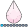## how to specify strata specific effects in PHREG?

How to i modify the following sample code to get the strata specific effects for a and b? thanks

proc phreg data = abc;

class a b;

model time*event(0) = a b;

strata c d;

run;

1 ACCEPTED SOLUTION

Accepted SolutionsJacobSimonsen
Barite | Level 11

## Re: how to specify strata specific effects in PHREG?

Hi Alexchien,

It is important to understand that the strata statement in PHREG means that the baseline hazard function can depend on the variables you put in the strata statement. The variables you put in the model statement will have same effect across the strata variables - unless ofcourse you have also putted the strata variables in the model line.

If you want the effect of A and B to depend of the level of C and D, then you can do as follows:

``````data simulation;
do i=1 to 1000;
A=rand('bernoulli',0.5);
B=rand('bernoulli',0.5);
C=rand('bernoulli',0.5);
D=rand('bernoulli',0.5);
t=rand('exponential',10);
output;
end;
run;

proc phreg data=simulation;
class A B C D/param=glm;
model t=A*C*D B*C*D;
hazardratio A/at(C=all D=all);
hazardratio B/at(C=all D=all);
run;

*or, if you want each strata to has its own baseline hazard function, you should also use the strata statement;

proc phreg data=simulation;
class A B C D/param=glm;
model t=A*C*D B*C*D;
strata C D;
hazardratio A/at(C=all D=all);
hazardratio B/at(C=all D=all);
run;``````

Notice that you need the "glm" parametrization to make the interactions effects that allows you to use C and D as effectmodifiers.

2 REPLIES 2JacobSimonsen
Barite | Level 11

## Re: how to specify strata specific effects in PHREG?

Hi Alexchien,

It is important to understand that the strata statement in PHREG means that the baseline hazard function can depend on the variables you put in the strata statement. The variables you put in the model statement will have same effect across the strata variables - unless ofcourse you have also putted the strata variables in the model line.

If you want the effect of A and B to depend of the level of C and D, then you can do as follows:

``````data simulation;
do i=1 to 1000;
A=rand('bernoulli',0.5);
B=rand('bernoulli',0.5);
C=rand('bernoulli',0.5);
D=rand('bernoulli',0.5);
t=rand('exponential',10);
output;
end;
run;

proc phreg data=simulation;
class A B C D/param=glm;
model t=A*C*D B*C*D;
hazardratio A/at(C=all D=all);
hazardratio B/at(C=all D=all);
run;

*or, if you want each strata to has its own baseline hazard function, you should also use the strata statement;

proc phreg data=simulation;
class A B C D/param=glm;
model t=A*C*D B*C*D;
strata C D;
hazardratio A/at(C=all D=all);
hazardratio B/at(C=all D=all);
run;``````

Notice that you need the "glm" parametrization to make the interactions effects that allows you to use C and D as effectmodifiers.

## Re: how to specify strata specific effects in PHREG?

thanks for the explanation.

Discussion stats
• 2 replies
• 1358 views
• 2 likes
• 2 in conversation